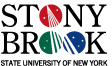MAT 667:
Spring 2012

## Topics covered

• Goldman bracket, definition and Jacobi identity (the Stone Alge4bra or a pair of pairs of points occuring twice), examples.
• One to one correspondance between free homotopy classes of oriented closed curves on a space X and conjugacy classes the fundamamental group of X.
• The number of terms of the Goldman bracket of two classes is a lower bound of their intersection number.
• "Following" intersection and self-intersection point of curves on a surface along a homotopy.
• Homework: The Goldman Lie algebra of curves in the torus.
• Equivariant homology of the free loop space of a free manifold (in degree 0 and 1) (equivariant with respect to the circle action rotating the domain)
• Homework: Let a and b denote the based homotopy classes corresponding to the boundary components of the pair of pant, oriented so that aB has self-intersection one. Consider the fundamental group of the pairs of pants with generators a, b. Compute the brackets, [aB, Ab] [aab, aB], [AAB, aB].
• The String Topology Lie algebra in the equivariant homology of the free loop space of a manifold (We will work out first on 3-manifolds and degree 0 and 1 equivariant homology).
• Classification of three manifolds.
• The String topology Lie algebra on a product of a surface with a circle. The String topology Lie algebra on Seifert fibered spaces.
• Several examples of 1-cycles in the equivariant homology of the free loop space of a free manifold.
• Brackets on the zeroeth and first equivariant homology groups of the free loop space of three manifolds.

## Tentative List of Topics and References

Below is a preliminary list of topics, not necessarily in the order they will be discussed.

• The Lie bialgebra of curves on surfaces
• Definition and examples. Goldman.
• The Goldman Lie algebra of curves in the torus.
• The Goldman Lie algebra of curves in a surface with boundary. Chas
• Relationship between the Goldman Lie algebra and formal symplectic geometry. Kawazumi- Kuno
• Digression: Self-intersections are Gaussian. Chas-Lalley
• The Goldman Lie algebra characterizes homeomorphisms. Gadgil,
• Intersection of and self-intersection number of curves and relation to the Goldman Lie algebra. Chas, Chas-Krongold
• The Lie bialgebra of curves on surfaces. Turaev
• Turaev's coalgebra and self-intersection of curves. Chas-Krongold, Le Donne.
• The center of the Goldman Lie algebra Etingoff,
• Goldman Lie algebra for Fuchsian groups
• Definition and examples.
• The Goldman Lie algebra on the Modular Surface.
• Intersection of geodesics on the Modular surface.
• String topology Chas-Sullivan
• Equivariant homology (of the free loop space) .
• A crash course on basic three manifold theory. Hatcher's notes. Also, the first section of the beautifully written Milnor's article. Finally, Brin's notes on Seifert fibered spaces
• Lie algebra on the free loop space of three manifolds. Definition and examples.
• The Lie algebra and the intersection number.
• The BV algebra structure on the homology of based loop space of a manifold.
• Basu
• Sullivan-Sullivan
• Detecting hyperbolicity of three manifolds with the loop product. Abbaspour .
• Andersen-Mattes-Reshetikhin algebra of chord diagrams Ellegaard Andersen, Mattes, Reshetikhin and Cahn's generalization's of Turaev's cobracket Cahn